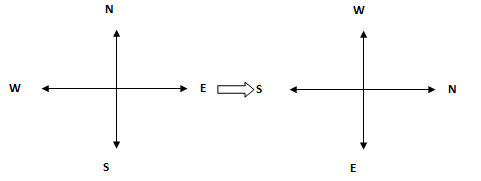# Crack SBI Clerk 2018 – Logical Reasoning (Inequality and Directions) Day-29

## Crack SBI Clerk 2018 – Logical Reasoning (Inequality and Directions) Day-29:

Dear Readers, Reasoning Section play a vital role in Banking and all other competitive exams. This year 2018 was started with three wonderful opportunities; Most Awaited SBI Clerk 2018. To enrich your preparation here we have providing new series of Practice Questions on Reasoning Ability – Inequality and Directions. Candidates those who are going to appear in Bank Exams 2018 can practice these questions daily and make your preparation effective.

[WpProQuiz 1487]

Click “Start Quiz” to attend these Questions and view Solutions

## Daily Practice Test Schedule | Good Luck

 Topic Daily Publishing Time Daily News Papers & Editorials 8.00 AM Current Affairs Quiz 9.00 AM Logical Reasoning 10.00 AM Quantitative Aptitude “20-20” 11.00 AM Vocabulary (Based on The Hindu) 12.00 PM Static GK Quiz 1.00 PM English Language “20-20” 2.00 PM Banking Awareness Quiz 3.00 PM Reasoning Puzzles & Seating 4.00 PM Daily Current Affairs Updates 5.00 PM Data Interpretation / Application Sums (Topic Wise) 6.00 PM Reasoning Ability “20-20” 7.00 PM English Language (New Pattern Questions) 8.00 PM General / Financial Awareness Quiz 9.00 PM

Directions (Q. 1-5): In these questions, a relationship between different elements is shown in the statement(s). The statements are followed by four conclusions. Give answer for the following questions

1. Statement: E > D = G ≤ H ≤ I = L

Conclusions:

1. L > D
2. D = L
3. E > I
4. L ≥ D
1.   None follows
2.   Only III follows
3.   Only IV follows
4.   Only either I or II and IV follows
5.   None of these
1. Statements: D > R < Y, V > W ≥ K = Y

Conclusions:

1. W > D
2. V ≤ Y
3. V > R
4. K ≥ R
1.   None follows
2.   Only III follows
3.   Only IV follows
4.   Only either III or IV follows
5.   None of these
1. Statements: U ≥ R = C, C ≥ N > M

Conclusions:

1. R > N
2. U > M
3. R ≥ M
4. N > U
1.   None follows
2.   Only I follow
3.   Only II follows
4.   Only III follows
5.   Only IV follows
1. Statement: Q > N ≥ R = B ≤ L < J

Conclusions:

1. J > R
2. Q ≥ L
3. Q > B
4. R > J
1.   Only I and III follow
2.   Only I and IV follow
3.   Only II and III follow
4.   Only II, III and IV follow
5.   None of these
1. Statement: L = B ≥ Z = U < P = R

Conclusions:

1. L ≥ U
2. Z < R
3. B > R
4. P > L
1.   Only I and III follow
2.   Only I and IV follows
3.   Only II and III follow
4.   Only II, III and IV follow
5.   None of these

Directions (Q. 6-7): Study the information carefully and answer the questions given below.

A man walks 8m towards east to reach point P. He turns to his right and walks 5m to reach point Q. Then, he turns to his left and walks 9m to reach point R and he turns to his right and walks 11m to reach point S. Finally he turns to his right and walks 20m to reach point T.

1. What is the distance between starting point and Q?
1.   13m
2.   9m
3.   √89m
4.   3√27m
5.   None of these
1. If a man walked 3m towards east from point T to reach point U, then what is the distance between point U and starting point?
1.   16m
2.   25m
3.   22m
4.   33m
5.   None of these
1. A person starts from point A, walks 5m towards south and reaches point B. He then turns left and walks 8m and reaches point C. He then takes a right turn and walks 6m. He takes a final right turn and walks 8m to reach point D. What is the distance between point C and D?
1.   13m
2.   12m
3.   10m
4.   15m
5.   None of these
1. A car started from point P and moves towards east. After moving a distance of 30m, it took a right turn, again after moving 15m, it took a left turn, and again after moving 10m, he took a right turn. Which direction is the car facing now?
1.   North
2.   South
3.   West
4.   North-west
5.   South-west
1. A directional post is erected on a crossing, in an accident it was turned in such a way that the arrow which was first showing east is now showing north. A passerby went in a wrong direction thinking it is east. In which direction is he actually travelling now?
1.   North
2.   East
3.   West
4.   South
5.   Cannot be determined

Directions (Q. 1-5):

1. E > D = G ≤ H ≤ I = L

L > D

D = L

E > I

L ≥ D follows

Either I or II and IV follows

1. D > R < Y, V > W ≥ K = Y

W > D not follows

V ≤ Y not follows

V > R follows

K ≥ R not follows

1. U ≥ R = C, C ≥ N > M

R > N not follows

U > M follows

R ≥ M not follows

N > U not follows

1. Q > N ≥ R = B ≤ L < J

J > R follows

Q ≥ L not follows

Q > B follows

R > J not follows

1. L = B ≥ Z = U < P = R

L ≥ U follows

Z < R follows

B > R not follows

P > L not follows

Directions (Q. 6-7):

1. Distance between starting point and Q =√(82+52)  = √(64+25)  = √89m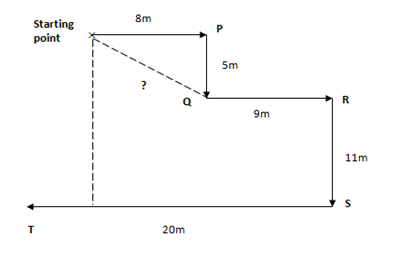1. Distance between U and starting point = 11 + 5 = 16m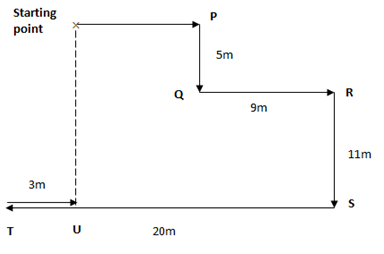1. Distance between C and D =√(82+62)  = √(64+36)  = √100 = 10m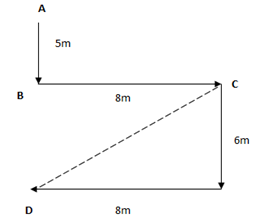1. The car is facing the south direction.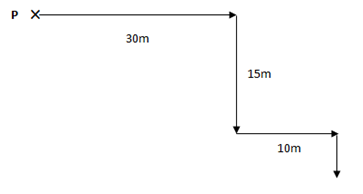1. When the arrow turns, north becomes west, east becomes north, south becomes east, west becomes south. So, he travels in south direction.